Practitioners lined up in rectangle with row with four, five or six exercisers, one always missing to full rectangle. How many exercisers were on the field, if they have estimated not been more than 100?

n =  59

### Step-by-step explanation: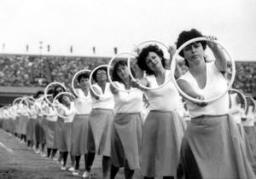Did you find an error or inaccuracy? Feel free to write us. Thank you!Tips to related online calculators
Do you want to calculate least common multiple two or more numbers?

## Related math problems and questions:

• Lcm simpleFind least common multiple of this two numbers: 140 175.
• LCM of two numberFind the smallest multiple of 63 and 147
• TilesHow many tiles of 20 cm and 30 cm can build a square if we have a maximum of 100 tiles?
• Game roomWinner can took win in three types of jettons with value 3, 30 and 100 dollars. What is minimal value of win payable in this values of jettons?
• Bouquet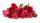Gardener tying bouquet of flowers for 8 and none was left. Then he found that he could tying bouquet of 6 flowers and also none was left. How many have gardener flowers (minimum and maximum) if they had between 50 and 100 flowers?
• Lcm of three numbersWhat is the Lcm of 120 15 and 5
• LCD 2The least common denominator of 2/5, 1/2, and 3/4
• LCMWhat is the least common multiple of 5, 50, 14?
• Summer camp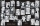Some boys or girls signed up for the summer camp, which has a maximum capacity of 200 children. The main leader noticed that during the evening start, he could arrange the participants exactly in the twelve-step, sixteen-step, or eighteen-step, and no one
• Dance groupThe dance group formed groups of 4, 5, and 6 members. Always one dancer remains. How many dancers were there in the whole group?
• School booksAt the beginning of the school year, the teacher distributed 480 workbooks and 220 textbooks. How many pupils could have the most in the classroom?
• Unknown integerFind the smallest integer: divided by 2, the remainder is 1. divided by 3, the remainder is 2. divided by 4, the remainder is 3. ... divided by eight, the remainder is 7, divided by 9 the remainder is 8.
• Sheep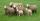Shepherd tending the sheep. Tourists asked him how much they have. The shepherd said, "there are fewer than 500. If I them lined up in 4-row 3 remain. If in 5-row 4 remain. If in 6-row 5 remain. But I can form 7-row." How many sheep have herdsman?
• Parachutists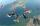Parachutists during freefall firstly held in groups of 4, then of 6, then 9, 12 and finally of 18 members. How many parachutists jump at least should be, if at each group must all be involved.
• Paving - jointsWe are paving with rectangular pavement 18 cm × 24 cm was placed side by side in height in a row and in the second row in width etc. How many times will the joints meet at a distance 10 m?
• PlumsIn the bowl are plums. How many would be there if we can divide it equally among 8, 10 and 11 children?
• The florist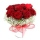The florist had 200 roses in the morning. During the day, more than half sold it. From the remaining roses, it will tie the bouquet. If a bouquet of 3, 4, 5, or 6 roses are bound, one always remains. How many roses from the morning shipment sold?